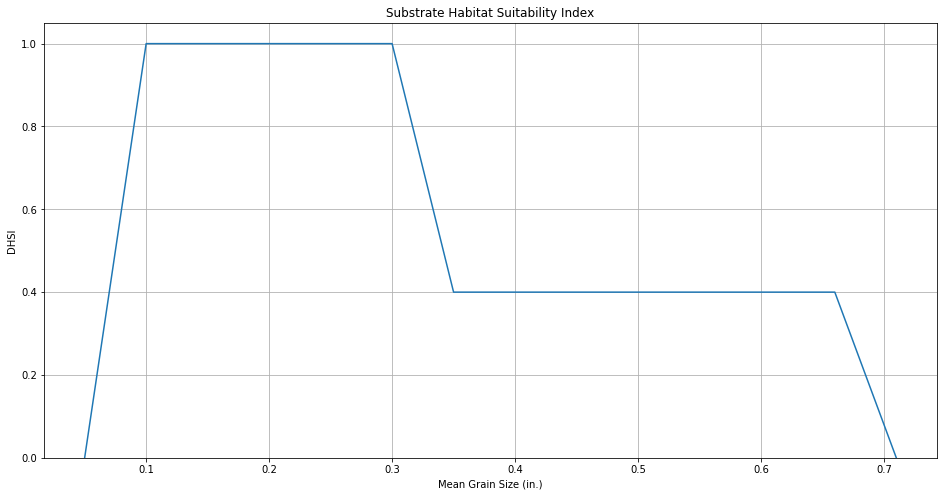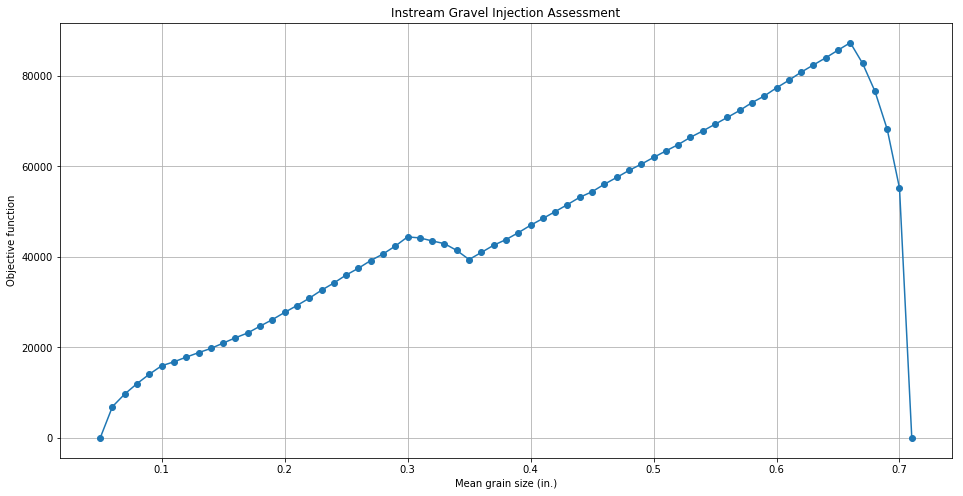#### Balancing ecology and morphodynamics for gravel augmentation

2020-01-22-river-substrate-optimization

## River Substrate Optimization: Balancing Habitat Benefit with Geomorphic Lifespan¶

Kenneth Larrieu

A vast range of factors with significant uncertainties must be considered when assessing salmonid habitat restoration projects in rivers. Here we will explore a simplified approach to probabilistic optimization of a habitat restoration project. The River Architect software described in Schwindt et al. (2019) estimates the cost of different restoration projects, the spatial distribution of stage-dependent salmonid habitat suitability throughout the modeled area, as well as the expected lifespan of the habitat areas (as they are subject to change via morphodynamic processes). A common restoration procedure in rivers which are deprived of sediment (e.g. due to damming) is gravel augmentation. The introduction of gravel and fine sediments into the river channel may enhance the suitability of salmonid habitat, however, once the sediment is transported downstream, the habitat suitability of the river reach may decrease again. Using larger gravel will increase the lifespan of the restoration features, but if the gravel is too large it will also be unsuitable for salmonid habitat. Therefore, one might want to optimize a gravel distribution throughout a river reach of interest by maximizing the habitat suitability and restoration feature lifespan. In this case, we will consider habitat suitability for spawning rainbow/steelhead trout in a reach of a California river downstream from a dam.

The habitat suitability of a parameter of interest can be quantified by a habitat suitability index ($HSI$), a function which ranges from $0$ to $1$, with $1$ indicating ideal habitat for the species. River Architect includes a module for calculating spatially explicit habitat suitability throughout a river reach. A composite habitat suitability index $cHSI$ is then taken as the geometric mean of $HSI$ values for all parameters of interest. In this application, the $cHSI(q, D)$ is taken as a function of depth and velocity (and in turn discharge $q$) as well as substrate size $D$:

$$cHSI = \left(hHSI \cdot uHSI \cdot DHSI\right)^{1/3}$$

where the prefixes $h$, $u$, and $D$ correspond to depth, velocity, and grain size.

The $DHSI$ used is plotted below:

In :
%matplotlib inline
import numpy as np
import matplotlib.pyplot as plt

def d_hsi(d):
"""substrate HSI for grain size d"""
if 0.05 <= d <= 0.1:
return (d-0.05)*1/0.05
elif 0.1 < d <= 0.3:
return 1
elif 0.3 < d <= 0.35:
return 1 - (d-0.3)*0.6/0.05
elif 0.35 < d <= 0.66:
return 0.4
elif 0.66 <= d <= 0.71:
return 0.4 - (d-0.66)*0.4/0.05
else:
return 0

fig, ax = plt.subplots(figsize=(16, 8))
ds = np.arange(0.05, 0.72, 0.01)
lines = ax.plot(ds, [d_hsi(d) for d in ds])
ax.grid()
ax.set(title='Substrate Habitat Suitability Index', xlabel='Mean Grain Size (in.)', ylabel='DHSI', ylim=[0, 1.05])
plt.show()Given the flood frequency distribution for the river reach, the lifespan of a restoration feature can be estimated as the recurrence interval of the corresponding flood at which the sediment becomes mobilized. Sediment is mobilized when the Shields stress (dimensionless bed shear stress) exceeds a critical value, taken to be $\tau^*_{cr} = 0.047$ (Lamb et al., 2008). Shields stress is computed as (Keulegan, 1938; Einstein, 1950):

$$\tau^* = \frac{1}{D_{84} g (s-1)} \left[\frac{u}{5.75 \log_{10}(12.2 h/(2 D_{84}))}\right]^2$$

where $u$ is the depth-averaged flow velocity, $h$ is the flow depth, $g$ is gravitational acceleration, $s$ is the ratio of sediment and water densities (taken to be $2.68$), and $D_{84}$ is the 84th percentile grain diameter. $D_{84}$ is estimated by multiplying the mean grain size by 2.2 according to Rickenmann and Recking (2011).

Using hydrodynamic model results for a given discharge, we can then calculate the spatially distributed maximum moblie sediment size. In other words, for a given discharge and location, this is the minimum grain size required for sediment stability. The maximum mobile sediment size at discharge $i$ is estimated as:

$$D_{mob, i} = \frac{h_i S_{e, i}}{(s-1)\tau^*_{cr}}$$

where $h_i$ and $S_{e,i}$ are the flow depth and energy slope, and $\tau^*_{cr}$ is the critical Shields stress.

We want to optimize the gravel augmentation for maximum habitat suitability and restoration feature lifespan. Therefore we might reasonably want to find the mean grain size $D$ which maximizes the product of $cHSI$ and lifespan $L$ summed over all pixels in the project reach:

$$\max_{D}\left(\sum_{pixels} \left[cHSI(q, D) \cdot L(D)\right]\right)$$

One of the parameters used that may have uncertainty is the critical Shields stress for sediment mobilization $(\tau^*_{cr})$. Based off the results of Lamb et al. (2008), we could reasonably guess the pdf $f(\tau^*_{crit})$ is normal with a mean of $0.047$ and standard deviation of $0.01$. Then, the optimization problem becomes:

$$\max_{D}\left(\sum_{pixels} \left[cHSI(q, D) \int_{-\infty}^{\infty} L(D, \tau^*_{crit}) f(\tau^*_{crit}) d\tau^*_{crit}\right]\right)$$

In addition, the flow $q$ for which the $cHSI$ is calculated should be the flow that occurs during the spawning season. Because the hydrodynamic model results are only available for discrete discharges, it is convenient to account for variability in the spawning season discharge by assigning probabilities to a range of discharges, and using the hydrodynamic model results for a single discharge as representative of that range. For the river reach of interest and considering the upstream release requirements during the spawning season for rainbow trout, the following table provides reasonable estimates for the probability of each spawning season discharge range:

$$\begin{array}{|r|r|} \hline q_i \text{ (cfs)} & p(q_i) \\ \hline 400-600 & 0.10 \\ \hline 600-800 & 0.25 \\ \hline 800-1,000 & 0.30 \\ \hline 1,000-2,000 & 0.35 \\ \hline \end{array}$$

Then, the optimization problem can be re-written as:

$$\max_{D}\left(\sum_{pixels} \left[ \sum_{q_i} \left[p(q_i) \, cHSI(q_i, D)\right] \int_{-\infty}^{\infty} L(D, \tau^*_{crit}) f(\tau^*_{crit}) d\tau^*_{crit}\right]\right)$$

This was achieved by making $hHSI$, $uHSI$, and stable grain rasters with River Architect, then applying the following code using ArcGIS's Python scripting capabilities:

In :
import os
import arcpy
from arcpy.sa import *

arcpy.CheckOutExtension("Spatial")
arcpy.env.overwriteOutput = True
path = os.path.join(os.getcwd(), "RA")

# inputs:
# high discharge file suffixes (indicate discharge in cfs)
l_suffixes = ['001k', '005k', '021k', '042k', '084k', '110k']
# recurrence interval for each high discharge
lifespans = [1.0, 1.2, 2.5, 4.7, 12.7, 20.0]
# stable grain size maps at each high/scouring discharge
grain_path = os.path.join(path, 'stable_grains')
stable_grain_maps = [Raster(os.path.join(grain_path, 'd_cr'+str(suffix))) for suffix in l_suffixes]

# spacing for tau_crit vals to integrate
dx = 0.001
# tau crits to integrate lifespan over
tau_crits = np.arange(0.017, 0.078, dx)
# mean/default tau crit
tau_crit0 = 0.047

# hydraulic HSI (product) at each low/spawning discharge
hsi_path = os.path.join(path, 'HSI')
s_suffixes = [530, 700, 880, 1000]
dsi_rass = [Raster(os.path.join(hsi_path, 'dsi_ras'+str(suffix))) for suffix in s_suffixes]
vsi_rass = [Raster(os.path.join(hsi_path, 'vsi_ras'+str(suffix))) for suffix in s_suffixes]
h_hsis = [dsi_rass[i] * vsi_rass[i] for i in range(len(s_suffixes))]
# probability of each spawning season flow range
ps = [0.1, 0.25, 0.3, 0.35]

# grain sizes to optimize
ds = np.arange(0.05, 0.72, 0.01)
# objective function value array
vals = []

def dot(l1, l2):
"""Dot product between two lists"""
return sum([e1 * e2 for e1, e2 in zip(l1, l2)])

def tau_crit_pdf(tau_crit, mu=tau_crit0, std=0.01):
"""pdf for tau crit"""
return 1.0/(np.sqrt(2*np.pi) * std) * np.exp(-(tau_crit - mu)**2 * 1.0/(2 * std**2))

# for each D_dist:
for d in ds:
# get expected cHSI:
c_hsis = [(d_hsi(d)*h_hsi)**(1.0/3) for h_hsi in h_hsis]
expected_cHSI = dot(ps, c_hsis)
# get expected lifespan:
expected_lifespan = 0
for tau_crit in tau_crits:
# adjust stable grain maps: multiply by old tau crit / new tau crit
adj_stable_grain_maps = [grain_map * tau_crit0/tau_crit for grain_map in stable_grain_maps]
# compare each pixel to stable grain maps for each discharge ---> create lifespan map (Con statements)
lifespan_map = Con(stable_grain_maps[-1] >= 0, 0, 0) # initialize map of zeros
for i in range(len(stable_grain_maps)):
lifespan_map = Con((stable_grain_maps[i] > d) & (lifespan_map == 0), lifespans[i], 0)
# multiply by pdf at the tau crit value
integrand = lifespan_map * tau_crit_pdf(tau_crit)
# multiply by dx and add to total integral value
expected_lifespan += integrand * dx

# take product of expected cHSI and Lifespan
expected_product = expected_cHSI * expected_lifespan
# sum all pixels
val = arcpy.RasterToNumPyArray(expected_product, nodata_to_value=0).sum()
# save value to array
vals.append(val)

# get maximum value
optimal_d, max_val = [(d, val) for d, val in zip(ds, vals) if val == max(vals)]
print('optimal grain size: %.2f' % optimal_d)

# plot objective function
fig, ax = plt.subplots(figsize=(16, 8))
ax.plot(ds, vals, '-o')
ax.set(title='Instream Gravel Injection Assessment', xlabel='Mean grain size (in.)', ylabel='Objective function')
ax.grid()
plt.show()

optimal grain size: 0.66Therefore, we see that the optimal mean grain size for gravel injection is $0.66 \text{ in}$. While this size is substantially larger than the range of $0.1-0.3 \text{ in.}$ indicating ideal spawning habitat, the larger grains are less mobile and the resulting increase in feature lifespan makes it preferable to use the larger grains.

Limitations and extensions:

Due to the complex nature of the problem, there are several areas where additional uncertainty may be incorporated for such an assessment. For example:

• The expected lifespans assume perfect knowledge of the flood frequency distribution.

• The $cHSI$ calculation assumes a constant discharge during spawning season. An extension might weight habitat suitability by the portions of spawning season for which particular discharges occur. In addition, the method of combining univariate HSIs via a geometric mean assumes independence of variables.

• The ratio of sediment and water densities used for the mobile sediment size distribution is also subject to uncertainty and spatial variability.

• While the hydrodynamic model results were validated against field data, there is uncertainty present in model outputs.

• Transport and deposition of sediment are not accounted for. A more explicit morphodynamic model could better constrain the lifespan of restoration features.

• The objective function used is somewhat arbitrary; for instance $cHSI$ may not be linearly proportional to more tangible habitat benefits such as salmon spawning rates.

• When gravel is scoured from the restoration site, it is transported downstream to other locations where it may still provide habitat benefit. This has not been accounted for.

Extensions could also incorporate cost objectives or make use of the relative economic utility provided by different levels of usable habitat.